# Optimal Tuning of Fractional Order Proportional-Integral-Derivative Controller for Wire Feeder System Using Ant Colony Optimization

Optimal Tuning of Fractional Order Proportional-Integral-Derivative Controller for Wire Feeder System Using Ant Colony Optimization

Noureddine HamoudaBadreddine Babes Cherif Hamouda Sami Kahla Thomas Ellinger Jürgen Petzoldt

Research Center in Industrial Technologies (CRTI), P.O. Box64, Cheraga 16014 Algiers, Algeria

ALCIOM Company, Yvelines, 3 rue des vignes 78220 Yvelines, France

Technisch Universität Ilmenau, Max-Planck-Ring 14, 98693 Ilmenau, Germany

Corresponding Author Email:
n.hamouda@crti.dz
Page:
157-166
|
DOI:
https://doi.org/10.18280/jesa.530201
9 January 2020
|
Accepted:
13 February 2020
|
Published:
20 May 2020
| Citation

OPEN ACCESS

Abstract:

The goal of this work is to present a robust optimal control approach, in order to improve the speed error-tracking and control capability of a permanent magnet DC Motor (PMDC) driven wire-feeder systems (WFSs) of gas metal arc welding (GMAW) process. The proposed speed controller employs an optimized fractional-order-proportional+integral+derivative (FOPID) controller that serves to eliminate oscillations, overshoots, undershoots and steady state fluctuations of the PMDC motor and makes the wire-feeder unit (WFU) has fast and stable starting process as well as excellent dynamic characteristics. The fixed controller parameters are meta-heuristically selected via an ant colony optimization (ACO) algorithm. Numerical simulations are performed in Matlab/Simulink environment and the performance of the proposed ACO-FOPID controller is validated. The simulation results clearly demonstrate the significant improvement rendered by the proposed approach in the wire-feeder system's reference tracking performance, torque disturbance rejection capability, and transient recovery time.

Keywords:

gas metal arc welding process, wire-feeder system, fractional-order-proportional-integral-derivative controller, ant colony optimization algorithm

1. Introduction

Nowadays, joining metals is a fundamental aspect of modern industrialized operations such as the ship building, automotive, and construction industries. It can be accomplished by different arc welding techniques. Among the various types of welding the typical GMAW is the most frequently employed and economically important welding process for joining metals. It is preferred for its flexibility, rapidity and can be utilized for both manual and automatic modes of welding for wide range of ferrous and non-ferrous metal pieces . Consistency and high-quality welding procedures are the key issues to maintain and increase the overall product quality.

During GMAW, the electrode wire is melted and liquid droplets are formed at the tip of the electrode. When detaching from the electrode, the droplets transfer both mass and heat into the weld pool. In order to achieve quality welds, the transfer process must be controlled. One of the strategies to control the quality of the weld is to maintain the set values of welding current and arc length to achieve the preferred values of heat and mass transfer to the work-piece. The control variables selected are, the wire feed rate and the open circuit voltage, which are utilized to control the current and arc length of the GMAW process . Large swing in wire feed rate results in large increased stress in PMDC motor and in welding current during welding process. This changes in wire feed rate causes the arc breaking, affecting the arc stability which ceases the welding operation. Therefore, wire-feeder system (WFS) is an important subsystem of typical automatic GMAW process. It should not only prevent the fracture and vibration of wire from occurring, but also guarantee the high speed of wire feed to meet the need of high rate of automatic welding production. Therefore, the control of wire feed rate for the WFS is the key technologies of the wire-feeder units (WFUs), which has a strong impact on the welding quality. The available WFUs are designed for constant wire feed rate and feature a large inertia and static friction due to the reduction gearbox and the eccentricities in the wire roller mechanism, and also due to the wire spool and the important frictions of the wire feed path . Thus, this mechanical dynamic is very slow as compared to the arc welding melting process .

Many solutions have been introduced in the literature to improve the wire feed speed responses of the WFUs, either by developing new mechanisms with various types of permanent magnet DC (PMDC) motors or by designing robust wire feeder controllers [4, 5]. The accurate design of wire feeder controller is essential to provide productivity, wide range welding capability, and comfort level to the welder user. One of the most widely used wire feed speed-regulation schemes is the proportional, integral and derivative (PID) controller . It is a simple and stable controller that provides a reliable control effort based on the weighted sum of the error-dynamics. The integral controller aids in eliminating steady-state errors and offers reasonable damping to attenuate overshoots, undershoots and persistent oscillations at the expense of the systems convergence rate. Meanwhile, the derivative controller improves the transitional times but injects high-frequency noise into the response. PI controllers are unable to compensate unprecedented state variations occurring in complex dynamical systems. Fuzzy controllers are difficult to synthesize because they require a lot of training data and elaborate logical rules to deliver robust control decisions .

Variable structure sliding mode controllers (SMCs) offer a robust control effort [8-10]. However, they inject chattering in the response and expend a lot of control energy. The augmentation of integer-order PID controllers with fractional calculus enables the control strategy to compensate for the effects of the un-modeled intrinsic nonlinearities associated with real-world dynamical systems . In this case, the classical integral operator is replaced by a fractional-order integral operator . The addition of a fractional-order parameter, along with the three PID controller gains, increases the degree of freedom and design flexibility of the controller .

However, the FOPID controllers may not provide the desired performance under changing operating conditions to the trial and error methods used to select and tune their gains parameters. Hence, various methods using heuristic optimization algorithms for parameters tuning of FOPID controller are available in the literature namely; genetic algorithm (GA) , artificial bee colony (ABC) algorithm , fuzzy logic algorithm (FLA) , particle swarm optimization (PSO) algorithm  and ant colony optimization (ACO) algorithm .

Jain et al.  introduced PSO for optimum design of FOPID controller in a PMDC motor speed control system. The simulation results demonstrate that the PSO algorithm improved the peak overshoot, rise time and settling time of a PMDC motor.

Gunawan et al.  presented an optimal approach to obtain the best values of the FOPID parameters using PSO and GA algortithms. The simulation results demonstrate that better control performance can be achieved using PSO algorithm in comparison with Nelder-Mead and genetic algorithm.

Singh et al.  presented a parameter optimization strategy for FOPID controller using ACO algorithm. The algorithm has been applied to the integer and fractional order plants and the results indicate high precision of control and quick response.

Kurniawan et al.  proposed a solution algorithm based on the cross entropy method (CEM) to determine the parameters of the FOPID controller for getting a well performance for a PMDC motor model system. The simulation results demonstrate that the FOPID-CEM controller has superior performance, both when compared with Ziegler-Nichols PID controller as well as other controllers which are tuned with the same CEM algorithm.

Due to its faster convergence and flexibility, an ant colony optimization (ACO) algorithm is adopted in this paper for the optimal adjustment of suggested FOPID controller. To the best of author’s knowledge, there is no study proposed in literature to improve the speed error-tracking and the control capability of WFSs with the optimal tuning FOPID controller. Based on previous consideration and analysis, the main contribution of this paper can be summarized as follows:

1. A combination of ACO algorithm with FOPID controller, namely the ACO-FOPID controller is proposed.
2. The proposed ACO algorithm is applied to the parameter tuning problem of FOPID controller for PMDC motor speed control of WFSs. This is the first application of FOPID controller and ACO algorithms in WFSs of GMAW process.
3. The performances of the proposed approaches are compared with other metaheuristic algorithms; namely the particle swarm optimization (PSO) and fuzzy logic optimization (FLO) algorithm in terms of the root mean square (RMS) values and the maximum (MAX) values of the sampled speed tracking error using the same objective function.
4. Comparative transient response and robustness analysis of the PMDC motor speed control system under the different operating conditions are carried out for the proposed ACO-FOPID controller.
5. Also, this paper investigates the more sensitive parameters for the FOPID controller.
2. Structure of GMAW Process

In the GMAW process, the welding inverter controls the open circuit voltage Voc(V) between the contact tip tube and the work-piece. In addition, the wire feed servo motor rotates a set of pinch rollers, which force the wire into the torch head and through the contact tube whereupon the wire is consumed by the GMAW process as illustrated in Figure 1.

## 1.jpg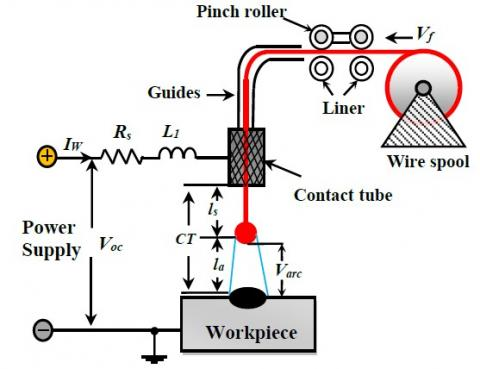Figure 1. Representation of WFS for GMAW process

The wire feed servo motor is in itself a feedback controlled system which is capable of delivering wire to the weld process at a controlled wire feed rate; increasing or decreasing the wire feed speed Vf(m/s) on the wire feeder servo motor increases or decreases the welding current Iw(A) as well as the metal transfer mode . In majority of cases, the value of Vf(m/s)) is reserved constant at desired value .

For the purposes of this derivation, the wire feed rate is considered to be the input. The arc dynamics form the plant larc(m) and the welding current IW(A) is taken to be the output.

The dynamic equation for the electrical circuit of the GMAW process, is given by Eq. (1).

${{V}_{oc}}=\,{{L}_{1}}\frac{d{{I}_{W}}}{dt}+{{R}_{s}}{{I}_{W}}+{{V}_{arc}}$   (1)

where, Voc(V) represents the open circuit voltage of arc welder power supply, Iw(A) is the instantaneous welding current, Rs(Ω) is the Thevenin resistance of arc welder power supply plus cabling resistance and L1(mH) is the inductance of arc welder power supply. The dynamic equation of arc voltage Varc(V) is expressed as :

${{V}_{arc}}=\,{{k}_{a}}{{l}_{arc}}+{{k}_{p}}{{I}_{W}}+{{V}_{c}}$   (2)

where, ka, kp, Ucare parameters of arc characteristics, and larc(m) is the arc length. The dynamic equation of arc length larc(m), is illustrated as Eq. (3):

$\frac{d l_{a r c}}{d t}=V_{m}-V_{f}$  (3)

where, Vm(m/s) represents the wire melting rate may be expressed as:

$V_{m}=k_{m} I_{W}$  (4)

where, km indicates the coefficient of wire melting rate. The dynamic equation of the power source Voc, is written as:

$V_{o c}=\left(R_{u}+k_{1} I_{W}\right) k_{0}$  (5)

where, Ru(V) is the control input of the power source, k0 is gain of power source and k1 is feedback gain.

3. Dynamics Model of PMDC Motor Driven WFSS

The graphic illustration of power circuit of the wire-feed servo motor control is depicted in Figure 2.

## 2.jpg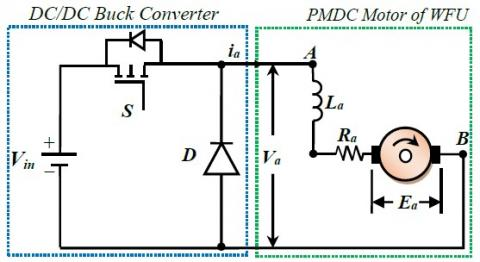Figure 2. Power circuit for the wire-feed servo motor control

The PMDC motor control variables are DC input voltage Va(V) and load torque TL(N.m). In WFUs, TL depends on diameter d(cm) of electrode wire and its material composition. For a particular application it could be considered as constant. The PMDC motor output variables are the wire feed speed Vf(m/s), the angular displacement of the motor shaft θ(rad) and the armature current ia(A).The way that section titles and other headings are displayed in these instructions, is meant to be followed in your paper.

The PMDC motor’s dynamic equations can be developed based on the Kirchhoff’s voltage law around the armature circuit and the Newton’s moment law using the Eqns. (6-8).

$V_{a}=L_{a} \frac{d i_{a}}{d t}+R_{a} i_{a}+k_{2} \frac{d \theta}{d t}$  (6)

$J \frac{d^{2} \theta}{d t}+f \frac{d \theta}{d t}=k_{3} i_{a}$  (7)

$V_{f}=k_{4} \frac{d \theta}{d t}$  (8)

where, Va(V) is the armature voltage, ia(A) is the armature current, Ra(Ω) is the armature resistance, La(mH) is the armature inductance, θ(rad) is the angular displacement of the motor shaft, Vf(m/s) is the wire feed rate, J(kg·m2) is the moment of inertia of the motor and mechanical load converted to the motor shaft, f(Nm·s) is the coefficient of viscosity of the motor and mechanical load converted to the motor shaft, k2, k3and k4 are constants.

Taking the Laplace transform of Eqns. (6-8), the configuration of the control unit of the PMDC motor can be shown as the block diagram in Figure 3.

## 3.jpg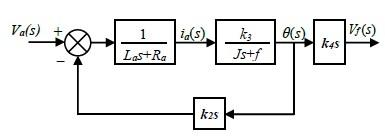Figure 3. Block diagram of wire-feed motor

According to Figure 3, the transfer function can be derived as:

$\frac{\theta(s)}{V_{a}(s)}=\frac{k_{3}}{s\left(L_{a} J s^{2}+\left(L_{a} f+R_{a} J\right) s+R_{a} f+k_{3} k_{2}\right)}$  (9)

If the motor inductance, La, is neglected, then

$\frac{\theta(s)}{V_{a}(s)}=\frac{k_{3}}{s\left(R_{a} J_{s}+R_{a} f+k_{3} k_{2}\right)}=\frac{k_{m}}{s\left(T_{m} s+1\right)}$  (10)

The gain of the wire-feed mechanism is

$K_{m}=\frac{k_{3}}{R_{a} f+k_{3} k_{2}}$  (11)

The time constant of the wire-feed mechanism is

$T_{m}=\frac{R_{a} J}{R_{a} f+k_{3} k_{2}}$  (12)

Therefore, the transfer function of the wire-feed mechanism may be simplified as

$\frac{V_{f}(s)}{V_{a}(s)}=\frac{K_{m} k_{4}}{T_{m} s+1}$  (13)

The control-block diagram of the regulated wire-feed rate system may be simplified as shown in Figure 4. from this last Rvf(s) represents the control input to the wire-feed motor and k5 is the open-loop gain of the motor circuit . According to Figure 4, the transfer function of the system and its dynamic characteristics can be derived as:

$\frac{L_{a}(s)}{R_{v f}(s)}=\frac{k_{5} K_{m} k_{4}}{T_{m} s^{2}+s+k_{5} K_{m} k_{a} k_{4}}$  (14)

$\zeta=\frac{1}{2 \sqrt{T_{m} k_{5} K_{m} k_{a} k_{4}}}$  (15)

$\omega_{n}=\sqrt{\frac{k_{5} K_{m} k_{a} k_{4}}{T_{m}}}$  (16)

$\omega_{d}=\sqrt{\frac{4 T_{m} k_{5} K_{m} k_{a} k_{4}-1}{2 T_{m}}} \approx \frac{\sqrt{k_{5} K_{m} k_{a} k_{4}}}{T_{m}}$ (17)

$\sigma=\zeta \omega_{n}=\frac{1}{2 T_{m}}$  (18)

In most instances, Tmk5KmKaK4 > 1 and 0 <ζ< 1; therefore, the system is in an under-damped state.

## 4.jpg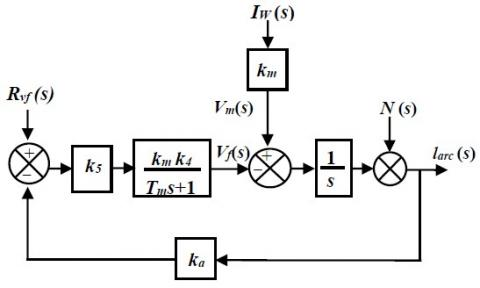Figure 4. Diagram of regulated wire feed-speed system

For simulation, the parameters and their values used in the present work for wire-feed motor control have been given in Table 1.

Table 1. Specifications of the Wire-Feed Motor

 Parameters Symbol Value Armature resistance Armature inductance Rotary inertia Viscous damping coefficient Back EMF constant Electromagnetic torque cst Ra La J F k2 k3 1.2 Ω 0.96 mH 1*10-4 kg.m2 1.29*10-3 .m.s/rad 0.0573 V.s/rad 0.0573 N.m/A DC input voltage Va 24V
4. Wire Feed Speed Controller Design

Generally, the wire feed speed controller is designed to realize accurate and robust optimal tracking of the preferred wire speed from no load to full load conditions. Hence, a simple ACO-FOPID control law is employed in this paper to achieve the design objectives as closely as possible.

4.1 Fractional calculus

The fundamentals of fractional calculus are described by three common definitions provided by Riemann-Liouville, Gruunwald-Letnikov, and Caputo . These definitions are outlined in Eqns. (19-21).

$G^{\beta} f(t)=\frac{1}{\Gamma(n-\beta)} \frac{d^{n}}{d t^{n}} \int_{a}^{t} \frac{f(\tau)}{(t-\tau)^{\beta-n+1}} d \tau$  (19)

where, Γ(x) represent the Euler gamma function, n is the integer number, and n-1< β < n.

$G^{\beta} f(t)=\lim _{h \rightarrow 0} \frac{1}{h^{\beta}} \sum_{j=0}^{(t-a) / h}(-1)^{j}\left(\begin{array}{c}\beta \\ j\end{array}\right) f(t-j h)$  (20)

where, $\left(\begin{array}{l}\beta \\ j\end{array}\right)=\Gamma(\beta+1) / \Gamma(j+1) \Gamma(\beta-j+1)$,

and h is the step-size.

$G^{\beta} f(t)=\frac{1}{\Gamma(\beta-n)} \int_{a}^{t} \frac{f^{n}(\tau)}{(t-\tau)^{\beta-n+1}} d \tau$  (21)

The conventional PI controller is transformed into the FO-PID controller using the definitions outlined above.

4.2 Fractional order PID controller

In 1994, Podlubny proposed the fractional PID controller and termed it PIλDµ controller. It had the fractional components λ and µ in integrator and differentiator respectively. Figure 5 shows the block diagram of Fractional-Order PID controller having input as error (e) and output (u).

## 5.jpgFigure 5. Fractional-order PID controller

The FO-PID control law, in the time-domain, is given by Eq. (22).

$u_{F O P I D}(t)=k_{p} e(t)+k_{i} D_{t}^{-\lambda} e(t)+k_{d} D_{t}^{\mu} e(t)$  (22)

The generalized transfer function of the FOPID control law is given as follows:

$\frac{U(s)}{E(s)}=k_{p}+k_{i} s^{-\lambda}+k_{d} s^{\mu}$  (23)

$G_{\text {FOPID}}(s)=k_{p}+k_{i} s^{-\lambda}+k_{d} s^{\mu},(\lambda, \mu>0)$  (24)

where, kp, ki and kd are proportional, integral and derivative gains constants, respectively, λ and µ are factional order of the integral and derivative term.

The term s in Eq. (24), has a fractional order that makes it difficult to implement. Hence, in this paper, the fractional integral operator is approximated using a 5th order Oustaloup’s recursive filter for the practical implementation of a FOPID controller in a digital computer. The Oustaloup’s approximation of sλ is given by Eq. (25):

$s^{\alpha} \approx K \prod_{n=-N}^{N} \frac{1+\left(\frac{s}{w_{z, n}}\right)}{1+\left(\frac{s}{w_{p, n}}\right)}, \alpha>0$  (25)

where, 2N+1 denotes the number of zeros and poles; K is the gain which causes both sides of Equation. $w_{z, n}$ and $w_{p, n}$ are given as:

$w_{z, n}=w_{b}\left(\frac{w_{h}}{w_{b}}\right)^{(n+N+1+(1-\alpha) / 2) /(2 N+1)}$  (26)

$w_{p, n}=w_{b}\left(\frac{w_{h}}{w_{b}}\right)^{(n+N+1+(1+\alpha) / 2) /(2 N+1)}$ (27)

In Eqns. (26) and (27), lower limit $w_{b}$ and upper limit wh normally satisfy wbwh=1 and k=wa h.

The case a<0 can be resolved by inverting Eq. (25). Besides, for ÷a÷>0, the approximation becomes unsatisfactory. In order to handle such case, the fractional powers of s is usually split, as follows:

$s^{\alpha}=s^{n} s^{\sigma}, \alpha=n+\sigma, \sigma \in[0.1]$  (28)

Hence, only the latter term needs to be approximated. The following Lemma 1 states the stability of fractional-order system.

Lemma 1. Consider the following autonomous system:

$_{0} \mathrm{D}_{t}^{\alpha}=C \ell, \ell(0)=\ell_{0}$  (29)

where, $\ell \in \Re^{n}$ and $C \in \Re^{n \times n}$ are asymptotically stable if $|\arg (\operatorname{eig}(C))|>\alpha \pi / 2$, in which each component of the states decays towards 0 like t-a.

Moreover, system (29) is stable if $\arg (\operatorname{eig}(C)) | \geq \alpha \pi / 2$ with those critical eigenvalues satisfying $|\arg (\operatorname{eig}(C))|=\alpha \pi / 2$ have geometric multiplicity one.

Besides, Figure 6 briefly shows the stability region when 0<a<2. It demonstrates that the stability region of fractional-order system with 0<a<1 is the largest than that of the other two scenarios.

FOPID controller involves tuning of five parameters: three parameters same as PID (kp, ki, kd) and two fractional parameters λ and µ. More flexibility is added in achieving the dynamics of control system by this expansion. In this work, the five fixed controller parameters of the FOPID control law are optimally tuned via an ant colony optimization (ACO) algorithm.

## 61.jpg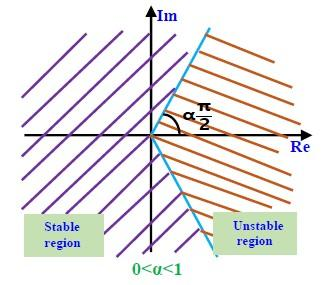## 62.jpg## 63.jpg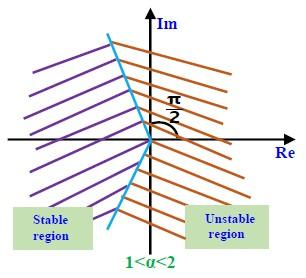Figure 6. Stability region of fractional-order system determined with varying operation order

4.3 Ant colony optimization (ACO) algorithm

Ant colony optimization algorithm is a meta-heuristic computational optimization technique, which deals with the nature inspired optimization method. It first brought out by Marco Dorigo [25, 26]. It is an indirect communication of ants in a colony with the help of pheromone trail. The behavior of ants can be explained as follow .

1. Firstly, each individual ant tries to find a solution for the specified optimization problem, the optimal solution is reached when the ants work as a colony.

2. While searching for the solution, ants interact indirectly by leaving the pheromone trail behind them.  Given a problem; each ant is allocated a state from where it commences its journey and moves to adjacent states in order to be able to identify the shortest path.

3. The path of the ant’s traverse depends upon the internal state; the pheromone trail and the information obtained from the surroundings.

4. Ants then release the pheromone and by the knowledge gained in the previous step; the quality of the path is judged by the amount of pheromone released

5. Finally, a pheromone matrix τ={τpq} is utilized by the ant system for obtaining an optimal solution. The starting state is defined as τpq = τo for all (p; q) where τo > 0. While releasing the pheromone when the ant moves from node p to node q; the probability is defined as:

$P_{p q}^{A}(t)=\frac{\left[\tau_{p q}(t)\right]^{\alpha}\left[\eta_{p q}(t)\right]^{\beta}}{\sum_{p, q \in T^{A}}\left[\tau_{p q}(t)\right]^{\alpha}\left[\eta_{p q}(t)\right]^{\beta}}$ (30)

where, τpq is the pheromone factor and ηpq= (l/dpq) is the Heuristic factor and dpq is the distance between nodes p and q. The pheromone factor comes from the knowledge and discovery made by the previous ants and the heuristic value is achieved from the particular problem being solved. α and β ≥ 0 are two constants determining the impact of the pheromone and heuristic factors on the decision of the ants. TY determines the route executed for a given time by the ant Y. The quantity of the pheromone $\Delta \tau_{p q}^{Y}$ on each iteration.

$\Delta \tau_{p q}^{Y}=\left\{\begin{array}{lc}\frac{L^{m i n}}{L^{Y}} & \text { if } p, q \in T^{Y} \\ 0 & \text { else }\end{array}\right.$  (31)

where, Lmin is the cost of the objective function discovered by the ant Y, and LY represents the optimal results found by all the ants until the present iteration.

## 7.jpg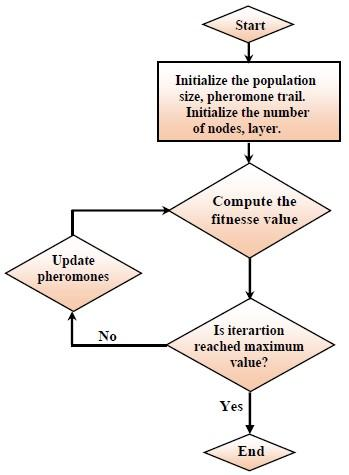Figure 7. Flowchart of ant colony optimization algorithm

In each iteration, the pheromones are updated by the expression:

$\sum \Delta \tau^{i}=\Delta \tau^{i=1}=\frac{\xi f_{b e s t}}{f_{\text {worst }}}$  (32)

The pheromone evaporation is a way to avoid unlimited increase of pheromone trails and is given by:

$\tau_{p q}(t)=\rho \tau_{p q}(t-1)+\sum_{Y=1}^{N Y} \Delta \tau_{p q}^{Y}(t)$  (33)

where, NY is the total number of ants and ρ determines the rate of evaporation of the pheromone (0<ρ<1), the flowchart of ant colony optimization algorithm is depicted in Figure 7.

4.4 Objective Function and constraints of the present work

The minimization of the integral of time-weighted absolute error (ITAE) criterion is adopted in this paper as an objective function. The ITAE objective function is given as:

$I T A E=\int_{0}^{t_{s i n}} t .|e(t)| . d t$  (34)

where, tsim is the simulation time and e(t) is the error signal that is the difference between reference wire feed speed Vf* and measured wire feed speed Vf,

$e=V_{f}^{*}-V_{f}$  (35)

In our case, the value of pheromone α is set to 1, the number of nodes is 1000, and the evaporation rate ρ is in the range from 0 to1, a value of 0.2 is chosen in this work. The block diagram of control system employing soft computing FOPID control action is shown in Figure 8.

## 8.jpgFigure 8. A block diagram of ACO-FOPID controller

When the ITAE objective function is minimized, the transient response of the PMDC motor wire feed speed control system is improved in terms of maximum overshoot, settling time and rise time.

The lower and upper limits of each FOPID controller parameters are: 0.001kp 20, 0.001≤ ki20, 0.001≤ kd ≤ 20, 0 ≤ λ ≤ 2 and 0 ≤ µ ≤ 2.

In Oustaloup’s approximation, ωL=10-3ωC, ωH=103ωC. where ωC is the transient gain frequency and the order of approximation N is 4.

5. Simulation Results and Discusions

The simulation program is built considering numerical values of wire-feed motor depicted in Table 1, the simulation results is carried out by using computer simulation program using Matlab/Simulink environment. The corresponding parameters of the tow controller are listed in Table 2.

Table 2. Parameters of ACO-PID, ACO-FOPID, Fuzzy-PID and PSO-PID controllers

 Controller kp ki kd λ µ ACO-PID 15.08 6.33 1.47 1 1 ACO-FOPID 11.77 9.12 1.04 0.82 0.94 Fuzzy-PID 10.07 4.85 0.86 0.61 0.33 PSO-PID 12.35 5.22 0.74 0.50 0.26

In this section, using Matlab/Simulation, the control performances and the effectiveness of the ACO-FOPID controllers are illustrated. For a fair comparison, the ACO algorithm designed for the FOPID is also applied to PID controller. The performances of the proposed ACO-FOPID controller are compared with other controllers such as ACO-PID controller, Fuzzy-PID controller and PSO-PID controller.

For this purpose, two different tests are applied, with the following changes for reference signals of speed wire:

In the first test, the reference signal for speed wire is chosen as a step curve of magnitude 24 cm/min, in order to evaluate the tracking control performance in continuous signals.

In the second test, the reference signal Vf* is chosen as stair curves with amplitudes of 8 cm/min, 16 cm/min and 24 cm/min in order to illustrate the tracking performance control of each controller under sudden change of Vf*.

5.1 Tracking performance of ACO-FOPID controller under step change of wire speed reference

## 9a.jpg(a) Tracking performance of Vf by ACO-FOPID controller

## 9b.jpg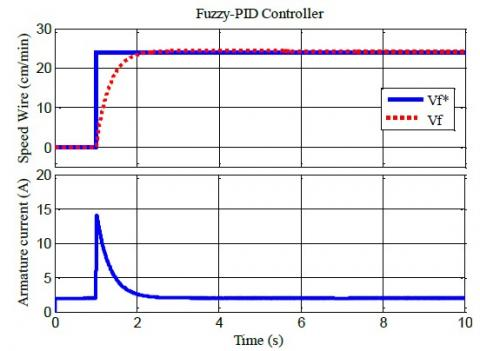(b) Performance tracking of Vf by Fuzzy-PID controller

## 9c.jpg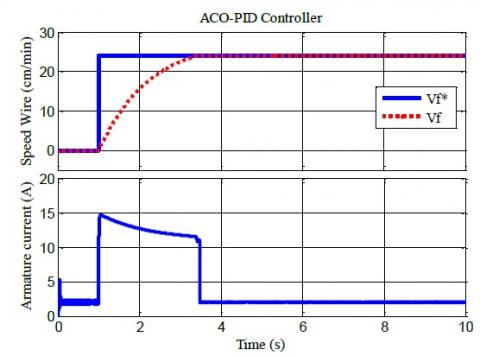(c) Tracking performance of Vf by ACO-PID controller

## 9d.jpg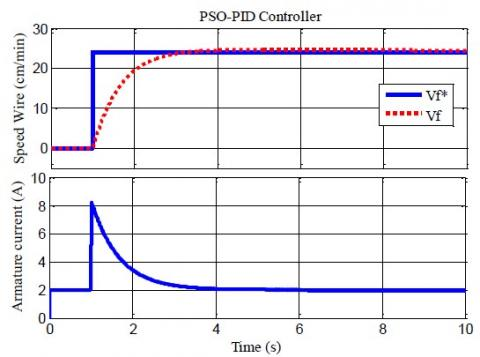(d) Tracking performance of Vf by PSO-PID controller

Figure 9. (a-d) Tracking performance of wire speed in the first test

Figure 9 (a-d) illustrates respectively the speed wire and the armature current of PMDC motor for each controller, at t=1s we apply a step change of wire speed reference from 0 cm/min to 24 cm/min is applied, Figure 9a shows that, when ACO-FOPID controller is used, the wire speed reaches quickly its reference value, with a delay of 1s, the starting armature current required to respond to this rapid change in speed is 15A. In comparing with other controllers such as: Fuzzy-PID, ACO-PID and PSO-PID controller, the ACO-FOPID controller presents good tracking control performances, in term of response time and the speed of convergence.

5.2 Tracking performance of ACO- FOPID controller when wire speed reference is a stair

Figures 10 (a-d) shows the tracking performances of wire speed obtained by using several controllers such as: ACO-FOPID controller, ACO-PID controller, Fuzzy-PID controller and PSO-PID controller, in this test, a stair curves are used as reference signal for wire speed, it increases and decreases progressively. It is noticed that best performances in tracking of wire speed are obtained with ACO-FOPID controller (Figure10a), the wire speed reaches rapidly its reference value, which justify the fast convergence and the short time response of the proposed controller against these changes, which are translated by good choice of optimal parameters of FO-PID by means of Ant colony optimization algorithm ( has good cost function), also the armature current of PMDC motor follows this variation, but it stays stable.

## 10a.jpg(a) Tracking performance of Vf by ACO-FOPID controller

## 10b.jpg(b) Tracking performance of Vf by ACO-PID controller

## 10c.jpg(c) Tracking performance of Vf by Fuzzy-PID controller

## 10d.jpg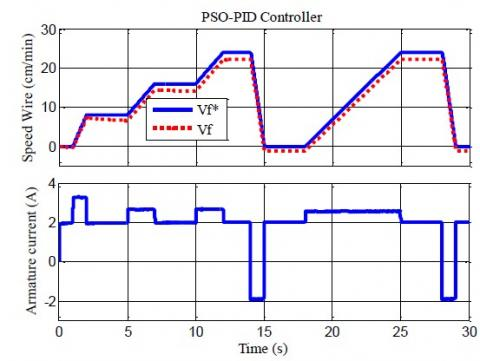(d) Tracking performance of Vf by PSO-PID controller

Figure 10. (a-d) tracking performance of wire’s speed in the second test

5.3 Evaluations the performance of the ACO-FOPID controller with others controllers

## 11.png(a) First test                                (b) Second test

Figure 11. MAX(e) of controllers for the two test

## 12.png(a) First test                                (b) Second test

Figure 12. RMS(e) value of controllers for the two tests

For more precise quantification of ACO-FOPID controller performances, two indexes are used quantify the sampled speed tracking error e(i): the RMS (Root Mean Square) and the maximum (MAX), they are defined as follows:

$R M S(e)=\sqrt{\sum_{i=1}^{N} \frac{e^{2}(i)}{N_{r}}}$  (33)

$M A X(e)=\operatorname{maximum}(|e|)$ (34)

It can be seen, from Figure 11 (a-b), that ACO-FOPID presents the best performances in terms of MAX(e), it has the smallest values between other controllers.

From the Figure 12 (a-b), the smallest root means square RMS(e) values obtained for each test are guaranteed using ACO-FOPID controller among the other controller.

6. Conclusions

In this paper, a suitable solution, to the problem of wire feed speed regulation in GMAW process, is introduced using a robust optimal FOPID control approach. The combination of ant colony optimization algorithm and FOPID controller yields better and robust control of wire feeder systems of welding machine. Numerical simulations are carried out in MATLAB/Simulink environment software and results presented and discussed. In each test, a comparison of performances obtained with ACO-FOPID and performances obtained with ACO-PID, Fuzzy-PID and PSO-PID controllers is carried out. Results shows that the optimized ACO-FOPID controller presents the best performances in terms of responses time and a good tracking, also an experimental validation of this project will be targeted by the authors in future work.

Acknowledgment

This work was supported by Research Center in Industrial Technologies (CRTI) and Directorate General of Scientific Research and Technological Development DGRST of Algeria.

References

 Naidu, D.S., Ozcelik, S., Moore, K.L. (2003). Modeling Sensing and Control of Gas Metal Arc Welding. Elsevier Publications, 1-8. https://doi.org/10.1016/B978-008044066-8/50003-3

 Kahla, S., Boutaghane, A., Letima, A., Dehimi, S., Hamouda, N., Babes, B., Amraoui, R. (2017). Grey wolf optimization of fractional PID controller in gas metal arc welding process. In Proc. 5th International Conference on Control Engineering and Information Technology CEIT, Sousse, Tunisia.

 Greene, B.W. (1988). Arc current control of a robotic welding system: Modeling and control system design. B.S., University of Illinois.

 Jiluan, P. (2003). Arc welding control. Woodhead Publishing Limited and CRC Press LLC © 2003, Woodhead Publishing Ltd.

 Naidu, D.S., Ozcelik, S., Moore, K.L. (2003). Modeling, Sensing and Control of Gas Metal Arc Welding. Elsevier Science Ltd. https://doi.org/10.1016/B978-0-08-044066-8.X5000-9

 Chaouch, S., Hasni, M., Boutaghane, A., Babes, B., Mezaache, M., Slimane, S., Djenaihi, M. (2018). DC-motor control using arduino-uno board for wire-feed system. Proceedings of the IEEE, 3rd CISTEM’18 Algiers, Algeria. https://doi.org/101109/CISTEM.2018.8613492

 Truong, D.Q., Ahn, K.K. (2009). Force control for hydraulic load simulator using self-tuning grey predictor–fuzzy PID. Mechatronics, Elsevier, 19(2): 233-246. https://doi.org/10.1016/j.mechatronics.2008.07.007

 Paul, A.K. (2014). Experimental design approach to explore suitability of PI and SMC concepts for power electronic product development. Int. J. Power Electronics, 6(1): 42-65. https://doi.org/10.1504/IJPELEC.2014.060707

 Ngo, M.D., Duy, V.H., Phuong, N.T., Kim, H.K., Kim, S.B. (2007). Development of digital gas metal arc welding system. Journal of Materials Processing Technology, 189(-3): 384-391. https://doi.org/10.1016/j.jmatprotec.2007.02.010

 Paul, A.K. (2019). Robust PMDC motor control for accurate wire feeding in GMAW using back EMF. IEEE Transactions on Industrial Electronics, https://doi.org/10.1109/TIE.2019.2896131

 Bingul, Z., Karahan, O. (2018). Comparison of PID and FOPID controllers tuned by PSO and ABC algorithms for unstable and integrating systems with time delay. Optimal Contr. Appl. Methods, 39(4): 1431-1450. https://doi.org/10.1002/oca.2419

 Tehrani, K.A., Amirahmadi, A., Rafiei, S.M.R., Griva, G., Barrandon, L., Hamzaoui, M., Rasoanarivo, I., Sargos, F.M. (2010). Design of fractional order PID controller for boost converter based on Multi-Objective optimization. in Proc. 14th IEEE PEMC, T3-179-T3-185, 2010. https://doi.org/10.1109/EPEPEMC.2010.5606514

 Verma, S.K., Yadav, S., Nagar, S.K. (2016). Fractional order PI controller design for non-monotonic phase systems. IFAC-Papers OnLine, 49(1): 236-240. https://doi.org/10.1016/j.ifacol.2016.03.059

 Samakwong, T., Assawinchaichote, W. (2016). PID controller design for electro-hydraulic servo valve system with genetic algorithm. Procedia Computer Science, 86: 91-94. https://doi.org/10.1016/j.procs.2016.05.023

 Yan, F., Wang, Y., Xu, W., Chen, B. (2018). Time delay control of cable-driven manipulators with artificial bee colony algorithm. Trans. Can. Soc. Mech. Eng., 42(2): 177–186. https://doi.org/10.1139/tcsme-2017-0043

 Kudinov, Y., Kolesnikov, V., Pashchenko, F., Pashchenko, A., Papic, L. (2017). Optimization of fuzzy PID controller’s parameters. Procedia Comput. Sci., 103: 618–622. https://doi.org/10.1016/j.procs.2017.01.086

 Bingul, Z., Karahan, O. (2018). Comparison of PID and FOPID controllers tuned by PSO and ABC algorithms for unstable and integrating systems with time delay. Optim. Control Appl. Methods, 39(4): 1431–1450. https://doi.org/10.1002/oca.2419

 Mavrovouniotis, M., Yang, S., Van, M., Li, C., Polycarpou, M. (2020). Ant colony optimization algorithms for dynamic optimization: A case study of the dynamic travelling salesperson problem. IEEE Computational Intelligence Magazine, 15(1): 52-63. https://doi.org/10.1109/MCI.2019.2954644

 Jain, R.V., Aware, M.V., Junghare, A.S. (2016). Tuning of fractional order PID controller using particle swarm optimization technique for DC motor speed control. 1st IEEE International Conference on Power Electronics. Intelligent Control and Energy Systems (ICPEICES-2016). https://doi.org/10.1109/ICPEICES.2016.7853070

 Gunawan, S.A., Yuwono, Y.C.H., Pratama, G.N.P., Cahyadi, A.I., Winduratna, B. (2018). Optimal fractional-order PID for DC motor: Comparison study. 4th International Conference on Science and Technology (ICST), Yogyakarta, Indonesia. https://doi.org/10.1109/ICSTC.2018.8528704

 Singh, R., Kumar, A., Sharma, R. (2016). Fractional order PID control using ant colony optimization. 1st IEEE International Conference on Power Electronics; Intelligent Control and Energy Systems (ICPEICES-2016). https://doi.org/10.1109/ICPEICES.2016.7853387

 Kurniawan, I., Cahyadi, A.I., Ardiyanto, I. (2018). Tuning fractional order proportional integral derivative controller for DC motor control model using cross-entropy method. 3rd International Conference on Information Technology, Information Systems and Electrical Engineering (ICITISEE), Yogyakarta, Indonesia. https://doi.org/10.1109/ICITISEE.2018.8721016

 Wu, G.D., Richardson, R.W. (1989). The dynamic response of self-regulation of the welding arc. In Proc. Recent Trends in Welding Science & Technology, Tennessee, USA, pp. 929-933. https://doi.org/10.5574/KSOE.2011.25.6.001

 Tepljakov, A. (2017). Fractional-order Modeling and Control of Dynamic Systems. Springer International Publishing. https://doi.org/10.1007/978-3-319-52950-9

 Dorigo, M., Caro, G.D., Gambardella, L.M. (1999). Ant algorithms for discrete optimization. Artificial Life, 5(2): 37-172. https://doi.org/10.1162/106454699568728

 Jinguo, S. (2019). A cost-effective pump scheduling method for mine drainage system based on ant colony otimization. Journal Européen des Systèmes Automatisés, 52(2): 123-128. https://doi.org/10.18280/jesa.520202

 Gokul, C., Vanchinathan, K., Gnanavel, C. (2019). Test scheduling of core based system-on-chip using modified ant colony optimization. Journal Européen des Systèmes Automatisés, 52(6): 599-605. https://doi.org/10.18280/jesa.520607